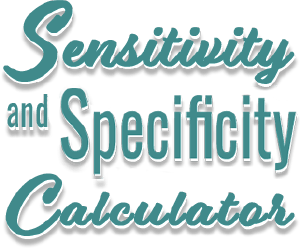We use cookies to provide you with a better experience. You can read our Cookie Policy here.

Stay up to date on the topics that matter to you# Sensitivity and Specificity calculator

When developing diagnostic tests or evaluating results, it is important to understand how reliable those tests and therefore the results you are obtaining are. By using samples of known disease status, values such as sensitivity and specificity can be calculated that allow you to evaluate just that.

What do sensitivity values tell you?

The sensitivity of a test is also called the true positive rate (TPR) and is the proportion of samples that are genuinely positive that give a positive result using the test in question. For example, a test that correctly identifies all positive samples in a panel is very sensitive. Another test that only detects 60 % of the positive samples in the panel would be deemed to have lower sensitivity as it is missing positives and giving higher a false negative rate (FNR). Also referred to as type II errors, false negatives are the failure to reject a false null hypothesis (the null hypothesis being that the sample is negative).

What do specificity measures tell you?

The specificity of a test, also referred to as the true negative rate (TNR), is the proportion of samples that test negative using the test in question that are genuinely negative. For example, a test that identifies all healthy people as being negative for a particular illness is very specific. Another test that incorrectly identifies 30 % of healthy people as having the condition would be deemed to be less specific, having a higher false positive rate (FPR). Also referred to as type I errors, false positives are the rejection of a true null hypothesis (the null hypothesis being that the sample is negative).

How do I calculate sensitivity and specificity values?

An ideal test rarely overlooks the thing you are looking for (i.e., it is sensitive) and rarely mistakes it for something else (i.e. it is specific). Therefore, when evaluating diagnostic tests, it is important to calculate the sensitivity and specificity for that test to determine its effectiveness.

The sensitivity of a diagnostic test is expressed as the probability (as a percentage) that a sample tests positive given that the patient has the disease.

The following equation is used to calculate a test’s sensitivity:

Sensitivity =                             Number of true positives

(Number of true positives + Number of false negatives)

=                         Number of true positives

Total number of individuals with the illness

The specificity of a test is expressed as the probability (as a percentage) that a test returns a negative result given that the that patient does not have the disease.

The following equation is used to calculate a test’s specificity:

Specificity =                     Number of true negatives

(Number of true negatives + number of false positives)

=                 Number of true negatives

Total number of individuals without the illness

Sensitivity vs specificity example

You have a new diagnostic test that you want to evaluate. You have a panel of validation samples where you know for certain whether they are definitely from diseased or healthy individuals for the condition you are testing for. Your sample panel consists of 150 positives and 400 negatives.

After running the samples through the assay, you compare your results to their known disease status and find:

True positives (test result positive and is genuinely positive)      = 144

False positive (test result positive but is actually negative)         = 12

True negatives (test result negative and is genuinely negative)   = 388

False negative (test result negative but is actually positive)        = 6

Or, displayed in a contingency table:

 Genuinely Positive Genuinely Negative Row Total Test Positive 144 12 156 Test Negative 6 388 394 Column Total 150 400 550

Sensitivity        = 144 / (144 + 6)

= 144 / 150

= 0.96

= 96 % sensitive

Specificity        = 388 / (388 + 12)

= 388 / 400

= 0.97

= 97 % specific